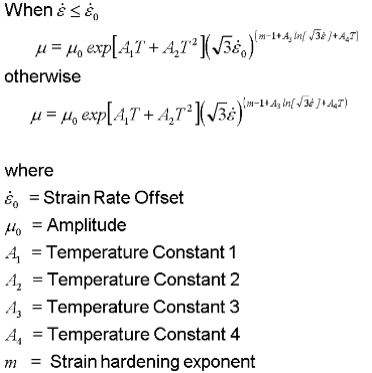﻿ Norton-Hoff Model
Manufacturing Solutions

# Norton-Hoff Model# Norton-Hoff ModelTemperature, strain, and strain rate dependent Norton-Hoff model used in HyperXtrude is given by the following expression.Workpiece Aluminum { ConstitutiveModel = "Norton-Hoff" Density = ρ SpecificHeat = Cp(T) Conductivity = K(T) CoeffOfThermalExpansion = βT VolumetricHeatSource = Qvol Amplitude = μ0 ConstantY = Y StressRateHardeningExponent = m ReferenceTemperature = T0 SolidusTemperature = Ts LiquidusTemperature = Tl TemperatureDependence = "Exp(A1T+A2T^2)" StrainOffset = ε TemperatureConstantA1 = A1 TemperatureConstantA2 = A2 TemperatureConstantA3 = A3 TemperatureConstantA4 = A4 YoungModulus = E PoissonRatio = ν}